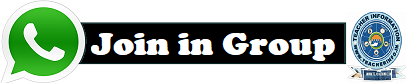## Wednesday, April 29, 2020

### SSC MATHS WORK BOOK FOR PRACTICE

1.REAL NUMBERS

Concepts
• The set of rational and irrational numbers together are called real numbers.
• The Fundamental Theorem of Arithmetic : Every composite number can be
• expressed (factorized) as a product of primes and this factorization is unique, apart
• from the order in which the prime factors occur.Oral questions

1. Define rational numbers?
2. Define Irrational numbers in two ways with examples?
3. State the fundamental theorem of arithmetic?
4. Are all integers also in real numbers? why?
5.Define logarithm?
6.. State the laws of logarithms?
7.. Is the sum or difference of a rational and an irrational is irrational?
8.. Is the product or quotient of a rational and an irrational is irrational?
9. The sum of two irrational numbers need not be irrational. Give an example?

---Prepared by: Allasubbarao, SA(Maths),8019312341.## TRENDING

✺ SCERT TEXT BOOKS CLASS 1 - 10